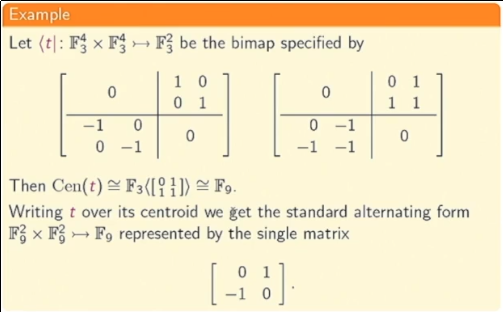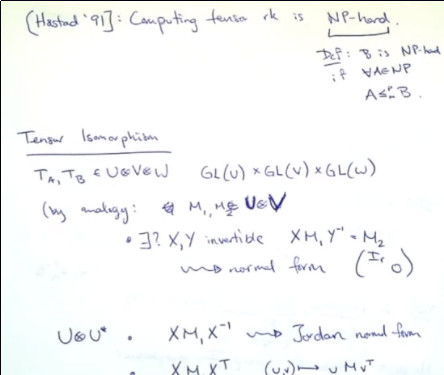# Algebraic Methods for Tensor Equivalence I & IIPeter Brooksbank

Speaker: Peter Brooksbank, Bucknell

Abstract: The purpose of this mini-course is to introduce algebraic tools that help to elucidate the structure of tensors. The focus will be on tensors of valence 2, or bilinear maps as they are also known. The main objects of study will be rings of matrices associated to a bilinear map that are invariant under isomorphism. These include commutative rings (centroids), rings with involution (adjoints), and Lie rings (derivations).

The motivation to study such rings comes from several directions. First, the structure and representation theory of these various types of rings is, to a greater or lesser extent, well understood. Secondly, that structure may in turn be used to decompose and study the associated bilinear map. Finally, since the defining conditions of these rings are all systems of linear equations, they can be constructed very efficiently from the given bilinear map.

Among the objectives of the mini-course are the following:

1. A survey of algorithms for computing with matrix algebras.
2. An analysis of algebraic methods to test equivalence of tensors––successes and outstanding challenges.
3. An introduction to software for computing with tensors and their associated algebras.

### Algebraic Method for Tensor Equivalence I

TACA 2019. For more visit https://TheTensor.Space/. Creative Commons 2.0 CC-ND 2019 Peter Brooksbank.

The second lecture will be about what tensors can tell us about computational complexity. We will see several ways - both direct and indirect - in which problems on tensors lie at the heart of lower bounds in complexity theory.

### Algebraic Method for Tensor Equivalence II

TACA 2019. For more visit https://TheTensor.Space/. Creative Commons 2.0 CC-ND 2019 Peter Brooksbank.

If you have additional questions, feel free to reach out to a maintainer / contributor on the contact page.

## Tensors: Algebra-Computation-App...

#### Tensor & Complexity I & II